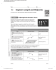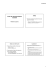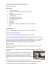Geometry Worksheet Name: Hodge

Transcription

Geometry Worksheet Name: Hodge
Geometry Worksheet
Name: ________________
Hodge-Podge over Constructions and Section 1.6
Period: _____
NOTE: This worksheet is WONDERFUL practice for part of TEST 1.2. I’d make sure you understand
EVERY problem. HINT, HINT, HINT!
I.
1.
196
2.
−5 52
4.
2 324
5.
( −6 3 )
II.
2
3.
(4 7 )
6.
10 252
2
Use the segments given to complete the requested constructions.
a
b
7. Construct a segment with length 2b + c .
9. Construct a segment with length
5
c.
2
c
8. Construct a segment with length 3b − c .
10. Construct a segment with length
1
3c − b .
2
II.
Use the angles given to complete the requested constructions.
x
y
11. Construct an angle with the measure
x+ z.
13. Construct an angle with the measure
III.
15. Find the coordinates of the midpoint
segment AB . SHOW YOUR WORK.
z
1
x.
2
12. Construct an angle with the measure
y−
2z .
14. Construct an angle with the measure
180 − y .
M of
16. E is the midpoint of segment DF . Given
points D and E , find the coordinates for point
A ( 6, −8 ) and B ( 9,18)


M

 ________, _________ 
D ( −8, −7 ) and E ( −16,10 )
F.


F

 ________, _________ 
IV.
17.
Find the distance
CD .
C (1,5 ) and D ( 5, −3)
CD = _______________
V.
STEP-IT-UP A NOTCH.
18. Find the perimeter of ∆ABC to the nearest tenth. You may use the distance formula or the
Pythagorean Theorem, but SHOW YOUR WORK.
AB = __________
CB = __________
AC = __________
Perimeter =
_______________
19. Find the length of each segment to determine
whether or not
AB ≅ CD .
C is the midpoint of AB . Point A has the
coordinates ( −6,8 ) and point B has the
20.
coordinates
( −6, −6 ) .
Find the distance from
C to
AB = ___________ CD = ___________
Is AB ≅ CD ? Why or why not?
CB = __________

Geometry Secondary Education MAFS.912.G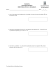The Midpoint Formula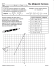Lesson 12: Dividing Segments Proportionately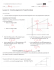pg. 485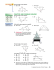Reteach Sec 1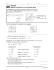STUDY GUIDE for Unit 1 Test Geometry 1. Z?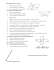Panel 2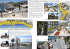TTCSI Services Talk final proposal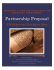Reteach Sec 1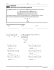Document 6510483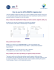PRODUCTS MARKETS Existing New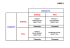CYCLE AND TENDENCY IN CIVIL CONSTRUCTION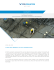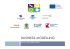Homework 5A: Green`s functions Math 456/556AVUTA Elections April 2015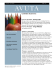Week of May 4 Lessons - Mr. Parr`s Science Class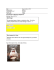Coordinate Geometry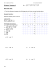HSM12CC_GM_06_09_CM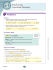Presentation Title - Masco Corporation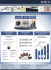1.1 Segment Length and Midpoints